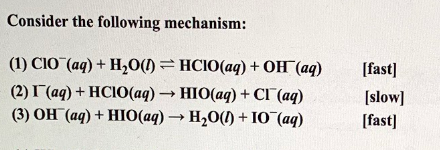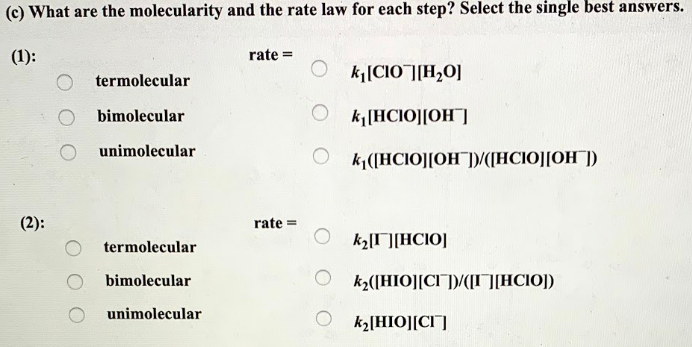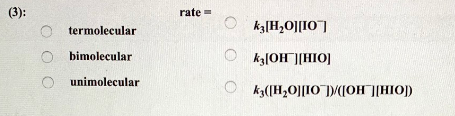# Consider the following mechanism: (1) ClO^-(aq) + H2O(l) ⇌ HClO(aq) + OH^-(aq) [fast] (2) I^-(aq) + HClO(aq) → HIO(aq) + Cl^-(aq) [slow] (3) OH^-(aq) + HIO(aq) → H2O(l) + O^-(aq) [fast] (c) What are the molecularity and the rate law for each step? Select the single best answers.